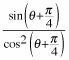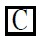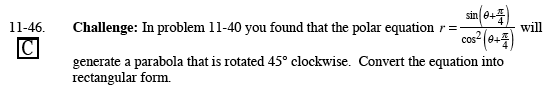Home > PC > Chapter 11 > Lesson 11.1.3 > Problem11-46

11-46.

In problem 11-40 you found that the polar equationwill generate a parabola that is rotated 45° clockwise. Convert the equation into rectangular form. Homework Help ✎Multiply both sides by cos²(θ + π/4).

Note: Steps can be done in various orders.

Expand sin(θ + π/4) and cos(θ + π/4) using the angle sum identities.

Change sin(π/4) and cos(π/4) to their numeric values.

Multiply both sides by r.

Substitute x for r cos(θ), etc.

Simplify.